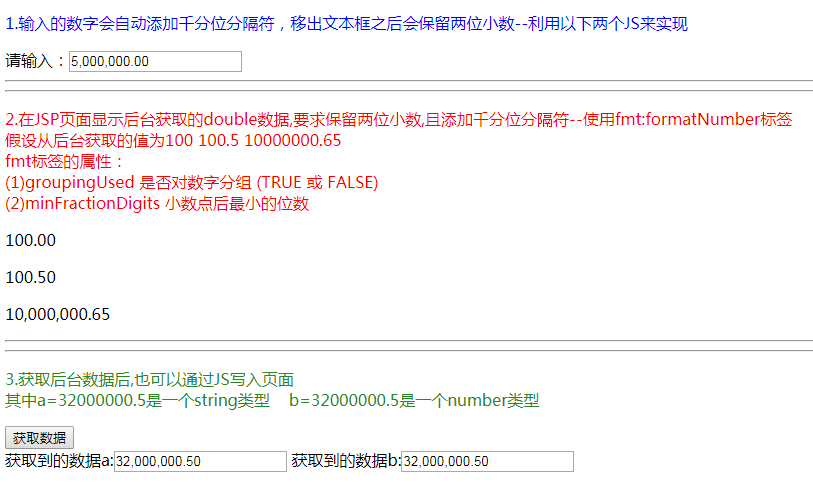• 1.新建filter.wxs ... return value.toFixed(2) // 此处2为保留两位小数，保留几位小数，这里写几 }, toStr: function (value) { return value.toString() }, toNum: function (value) {
1.新建filter.wxs
var filters = {

toFix: function (value) {

return value.toFixed(2) // 此处2为保留两位小数，保留几位小数，这里写几

},

toStr: function (value) {

return value.toString()

},

toNum: function (value) {

return value.toNumber()

},

}

module.exports = {

toFix: filters.toFix,

toStr: filters.toStr,

toNum: filters.toNum,//暴露接口调用

}
2.WXML中引入WXS(注意,该路径是我自己的路径)
<wxs module="filters" src="../../../../../utils/str.wxs"></wxs>
3.在WXML中使用
 {{ filters.toFix(price) }}

展开全文• 1.保留小数点后几 .toFixed() 出现使用原因：在小程序中 19.9 * 3 = 59.699999999999996，当然这个不是我们想要的值，因此我们需要四舍五入， 59.69999999996.toFixed(1) == 59.7 59.69999999996.toFixed(2) == 59...
1.保留小数点后几位 .toFixed()
出现使用原因：在小程序中 19.9 * 3 = 59.699999999999996，当然这个不是我们想要的值，因此我们需要四舍五入，
59.69999999996.toFixed(1)  == 59.7
59.69999999996.toFixed(2)  == 59.70
59.644.toFixed(2)  == 59.64


返回string 类型！

2.获取几位数 toPrecision()
类似向下取整。
3.125.toPrecision(1)    ==   3
3.125.toPrecision(2)    ==   3.1
3.125.toPrecision(3)    ==   3.12
3.125.toPrecision(4)    ==   3.125

展开全文toFixed
• 对于数据是浮点数类型的，有种方法（以保留小数点后三为例） 第一种方法： 保留几toFixed()参数就填几 numClick:function(){ var data = 0.79206174612045; console.log(data.toFixed(3)); } 第二种方法: ...
对于数据是浮点数类型的，有两种方法（以保留小数点后三位为例） 第一种方法： 保留几位toFixed()参数就填几
numClick:function(){
var data = 0.79206174612045;
console.log(data.toFixed(3));
}

第二种方法: 保留两位只需把1000改为100即可，以此类推
numClick:function(){
var data = 0.79206174612045;
console.log(Math.round(data*1000)/1000);
}

对于数据是字符串类型的： 保留n位小数通用公式是data.substring(0,data.indexOf(".")+(n+1))
numClick:function(){
var data = "0.79206174612045";
console.log(data.substring(0,data.indexOf(".")+4));
}

展开全文js
• 页面显示如下： ------------------------------------------------- ... 1.输入的数字会自动添加千分位分隔符，移出文本框之后会保留两...在JSP页面显示后台获取的double数据,要求保留两位小数,且添加千分位分隔
页面显示如下：-------------------------------------------------

<%@ page contentType="text/html; charset=UTF-8"%>
<%@ taglib uri="http://java.sun.com/jsp/jstl/fmt" prefix="fmt"%>

<!DOCTYPE html PUBLIC "-//W3C//DTD HTML 4.01 Transitional//EN" "http://www.w3.org/TR/html4/loose.dtd">
<html>
<meta http-equiv="Content-Type" content="text/html; charset=UTF-8">
<title>Insert title here</title>
<body>
<p style="color:blue">1.输入的数字会自动添加千分位分隔符，移出文本框之后会保留两位小数--利用以下两个JS来实现</p>
请输入：<input type="text" id="count"/>
<hr><hr>

<p style="color:red">
2.在JSP页面显示后台获取的double数据,要求保留两位小数,且添加千分位分隔符--使用fmt:formatNumber标签<br>
假设从后台获取的值为100 100.5 10000000.65 <br>
fmt标签的属性：<br>(1)groupingUsed  是否对数字分组 (TRUE 或 FALSE)<br>  (2)minFractionDigits	小数点后最小的位数
</p>
<p><fmt:formatNumber groupingUsed="true" minFractionDigits="2">100</fmt:formatNumber></p>
<p><fmt:formatNumber groupingUsed="true" minFractionDigits="2">100.5</fmt:formatNumber></p>
<p><fmt:formatNumber groupingUsed="true" minFractionDigits="2">10000000.65</fmt:formatNumber></p>
<hr><hr>
<p style="color:#228B22">3.获取后台数据后,也可以通过JS写入页面<br>
其中a=32000000.5是一个string类型    b=32000000.5是一个number类型
</p>
<button type="button" οnclick="getMsg()">获取数据</button><br>
获取到的数据a:<input type="text" id="msg1"/>
获取到的数据b:<input type="text" id="msg2"/>

</body>

<%
String basePath = request.getContextPath();
%>
<script type="text/javascript" src="<%=basePath%>/js/jquery-1.9.1.min.js"></script>

<script type="text/javascript">
var a = "32000000.5";//string类型
var b = 32000000.5;//number类型
//将a和b写入文本框
function getMsg(){
$("#msg1").val(toDoubleThousands(a));$("#msg2").val(toDoubleThousands(b));
}

//保留两位小数以及千分位的分隔符
function toDoubleThousands(num) {//可以传入number或者string
//判断是否有小数点
var s = num.toString().indexOf(".");
if(s == -1){//是整数
return (num || 0).toString().replace(/(\d)(?=(?:\d{3})+$)/g, '$1,')+".00";
}else{//是小数
var arr = num.toString().split(".");
if(arr.length > 1 && arr.length < 2){//一位小数
return (arr || 0).toString().replace(/(\d)(?=(?:\d{3})+$)/g, '$1,') + "." + arr + "0";
}else{//两位小数
return (arr || 0).toString().replace(/(\d)(?=(?:\d{3})+$)/g, '$1,') + "." + arr;
}
}
}

//千分位的分隔符
function toThousands(num) {//此时传入的是字符串,若传入double类型则会indexOf报错
//若没有任何数据则直接返回
if(num == "" || num == null){
return num;
}else{
//判断是否有小数点
var s = num.indexOf(".");
if(s == -1){//是整数
return (num || 0).toString().replace(/(\d)(?=(?:\d{3})+$)/g, '$1,');
}else{
var arr = num.split(".");
return (arr || 0).toString().replace(/(\d)(?=(?:\d{3})+$)/g, '$1,') + "." + arr;
}
}
}
//小数点的校验
$(function(){ //当按下按键时触发keyup事件$("#count").on('keyup', function (event) {
var $amountInput =$(this);
//响应鼠标事件，允许左右方向键移动
event = window.event || event;
if (event.keyCode == 37 | event.keyCode == 39) {
return;
}
//先把非数字的都替换掉，除了数字和.
var temp = $amountInput.val().replace(/[^\d.]/g, ""). //只允许一个小数点 replace(/^\./g, "").replace(/\.{2,}/g, "."). //只能输入小数点后两位 replace(".", "$#$").replace(/\./g, "").replace("$#$", ".").replace(/^(\-)*(\d+)\.(\d\d).*$/, '$1$2.$3'); //添加分隔符$amountInput.val(toThousands(temp));
});
//鼠标移出事件--保留两位小数
$("#count").on('blur', function () { var$amountInput = $(this); //最后一位是小数点的话，移除$amountInput.val(($amountInput.val().replace(/\.$/g, "")));
//保留两位小数
var s = $amountInput.val(); var arr = s.split("."); if(s == ""){$amountInput.val("0.00");
return;
}else if(arr.length == 1){//没有小数点
$amountInput.val(s + ".00"); return; }else if(arr.length > 1 && arr.length < 2){//有小数点$amountInput.val(s + "0");
return;
}
});
});
</script>
</html>



展开全文• input输入框输入数字时只能输入小数点两位通过正则验证保证，方法如下： moneyInput(e) { var money; if (/^(\d?)+(\.\d{0,2})?$/.test(e.detail.value)) { //正则验证，提现金额小数点后不能大于两位数字 ...input • 原理：wxml中不能直接使用较高级的js语法，如‘.toFixed’，‘toString()’，但可以通过引入wxs模块实现效果 1.新建filter.wxs ...return value.toFixed(2) // 此处2为保留两位小数，保留几位小数，这里写... • 保留小数点两位，但同时不要小数点后多余0的前后台代码实现 url：http://www.cnblogs.com/waterforever/archive/2010/03/25/1695289.html 今天碰到一个需求。关于小数点的处理，看起来非常简单的事情，却花了一定...javascript java 数据库 url • wxml中不能直接使用较高级的js语法，如.toFixed，但可以通过... return Number(value).toFixed(2)//此处2为保留两位小数 } } module.exports = { toFix: filters.toFix } WXML中引入WXS 并使用 <wxs module=. • 微信小程序开发交流qq群 173683895 承接微信小程序开发。扫码加微信。 正文： 主要作用域数值显示；请看源码： <!DOCTYPE html> <html> <head> <meta charset="utf-8"> </head... • 遇到的问题： 从后端传过一个二维数组 我的数据是空的，所以显示为0。 客户数据： ...小程序端修改代码也遇到问题了。...当时用toFixed这个方法一直报错...toFixed（2）,意思是小数点两位。 直接打印，可以了。... • input内强制保留小数点两位 位数不足时自动补0 小数点后位数超出2位时进行四舍五入 需引入jquery包 1.11.2版本 1 function xiaoshu(x) 2 { 3 var f = parseFloat(x); 4 var f = Math.round(x*100)/100... • 近来用ASP程序处理一些数据表单，遇到数字格式化的问题，如数据保留小数等问题，经过研究，可以使用ASP的内置函数FormatNumber来实现，下面就具体说说FormatNumber函数使用方法。 FormatNumber函数：返回格式... • 这篇文章主要介绍了ASP中保留小数点两位数的方法,使用FormatNumber函数实现,需要的朋友可以参考下 近来用ASP程序处理一些数据表单，遇到数字格效果可以看下具体51搜索展示http://www.51bt.cc，结合Xunsearch全文...全文检索 服务器 • 格式化浮点数的问题，用format（col,2）保留两位小数点，出现一个问题，例如下面的语句，后面我们给出解决方法 SELECT FORMAT(12562.6655,2); 结果：12,562.67 查看文档：Formats the number X to a format... • 有时调试程序时，我们可能想查看某些变量或者成员的地址，由于地址或者指针也不过是个32 的数，你完全可以使用打印无符号整数的”%u”把他们打印出来： sprintf(s, "%u", &i); 不过通常人们还是喜欢使用16 ... •$/.test(e.detail.value)) { //正则验证，提现金额小数点后不能大于两位数字 money = e.detail.value; } else { money = e.detail.value.substring(0, e.detail.value.length - 1); } this.setData({ money
• 最近在做一个项目，需要金额，但是如果在初始data设置价格为0.00则会在显示为0,今天特意记录一下方法 .toFixed(2) 特别注意一下toFixed()返回字符串，可以用parseFloat...欢迎大家开发程序多多交流tpwe8888 ...
• 今天写了一个小程序，Java 语言的测试BMI【谁不知道“BMI”的自行百度不解释】，涉及到了四舍五入并保留小数点两位，就总结了一下。先给大家看看程序 　 就是说给大家提供4种方法来保留小数点两位数 　//将算...
• 1.保留小数点后几小数，直接用.toFixed()即可.括号内的数字表示保留的位数.如果是整数,就会不上0， 2.获取几数 toPrecision() 方法一：指定要保留的小数位数(0.1+0.2).toFixed(1) = 0.3;这个方法toFixed是...
• 是将数字按宽度为2，采用右对齐方式输出，如果数据位数不到2，则左边补空格 %02d（0填充，右对齐） 默认情况下，数据数据宽度不够2是用空格填补的，但是因为2d前面有0，表示，数据宽度不足时用0填补。 %....
• 1，新建一个wxs文件 var numberUtil = { numberFormat: function (value) { var v = parseInt (value) //强转Int，毕竟有可能返回是String类型的数字...3、4什么的，toFixed(2)的参数可以动态传toFixed wxs
• 题目是这样的，已知底数n，请准确地计算出结果a = （），结果保留小数点后x。π是圆周率，可取值为3.141592653589793238462643383279502884197169399375。 输入格式: 第一行是一个整数t，表示测试实例的个数； ...控制输出小数位数
• 微信小程序input只能输入小数点前8，后2的正则验证 代码如下： //获取充值金额 btnInput: function(e) { var that = this; var mesValue //正则验证，充值金额仅支持小数点前8位小数点后2 if (/^\d{1,...input 正则验证
• Number.toPrecision(2);functiontoPrecision( [precision : Number] ) : String参数precision...备注对于以指数记数法表示的数字，将返回小数点后的precision - 1数字。对于以定点记数法表示的数字，将返回precisi......